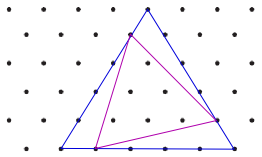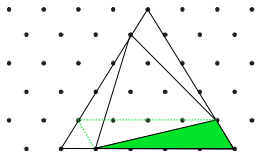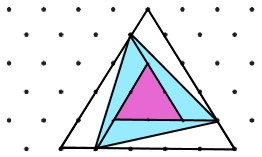#### You may also like### At a Glance

The area of a regular pentagon looks about twice as a big as the pentangle star drawn within it. Is it?### Six Discs

Six circular discs are packed in different-shaped boxes so that the discs touch their neighbours and the sides of the box. Can you put the boxes in order according to the areas of their bases?### Equilateral Areas

ABC and DEF are equilateral triangles of side 3 and 4 respectively. Construct an equilateral triangle whose area is the sum of the area of ABC and DEF.

# Of All the Areas

##### Age 14 to 16Challenge Level

You may find it useful to take a look at More Isometric Areas.
These diagrams might help you to work out the areas of the tilted triangles.

The top diagrams show how drawing a non-tilted triangle around the tilted triangle could help you to find the area of the tilted triangle.Area of tilted triangle = Area of blue triangle - Area of 3 green triangles

This diagram show some lines that can be drawn inside the tilted triangle:Area of tilted triangle = Area of pink triangle + Area of 3 blue triangles